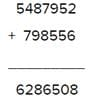Test: Addition and Subtraction- 1

# Test: Addition and Subtraction- 1

Test Description

## 10 Questions MCQ Test Mathematics for Class 4 | Test: Addition and Subtraction- 1

Test: Addition and Subtraction- 1 for Class 4 2022 is part of Mathematics for Class 4 preparation. The Test: Addition and Subtraction- 1 questions and answers have been prepared according to the Class 4 exam syllabus.The Test: Addition and Subtraction- 1 MCQs are made for Class 4 2022 Exam. Find important definitions, questions, notes, meanings, examples, exercises, MCQs and online tests for Test: Addition and Subtraction- 1 below.
Solutions of Test: Addition and Subtraction- 1 questions in English are available as part of our Mathematics for Class 4 for Class 4 & Test: Addition and Subtraction- 1 solutions in Hindi for Mathematics for Class 4 course. Download more important topics, notes, lectures and mock test series for Class 4 Exam by signing up for free. Attempt Test: Addition and Subtraction- 1 | 10 questions in 15 minutes | Mock test for Class 4 preparation | Free important questions MCQ to study Mathematics for Class 4 for Class 4 Exam | Download free PDF with solutions
 1 Crore+ students have signed up on EduRev. Have you?
Test: Addition and Subtraction- 1 - Question 1

### P = 4587946, Q = 5432322, R = 4595566. Find the value of P + Q − R.

Detailed Solution for Test: Addition and Subtraction- 1 - Question 1

P + Q - R = 4587946 + 5432322 - 4595566 = 5424702

Test: Addition and Subtraction- 1 - Question 2

### A + B + 45896 = C + D. lf C + D = 96023 + B, find the value of A.

Detailed Solution for Test: Addition and Subtraction- 1 - Question 2

It is given that C + D = A + B + 45896
According to the question
45896 + A + B = 96023 + B
45896 + A = 96023 + B - B
A = 50127

Test: Addition and Subtraction- 1 - Question 3

### You have to replace the digit 8 in the numbers 5487952 and 798556 such that their sum becomes 6231508. By which one of the following digits would you like to replace 8?

Detailed Solution for Test: Addition and Subtraction- 1 - Question 3We need to replace 8 by some digit to get Sum 6231508
6286508 - 6231508 = 55000
=> Sum would be reduced by 55000 Currently Values of 8 are 80000  & 8000
=> 88000 - 55000 = 33000 = 30000 + 3000
Hence 8 should be replaced by 3.

Test: Addition and Subtraction- 1 - Question 4

What is the difference between the place value of 2 and 4 in the number 23457.

Detailed Solution for Test: Addition and Subtraction- 1 - Question 4

Place value of 2 is 20,000
Place value of 4 is 400
Therefore, the difference between 20000 & 400 is 19600

Test: Addition and Subtraction- 1 - Question 5

What greatest number should be subtracted from 2546987 such that the difference becomes divisible by 3?

Detailed Solution for Test: Addition and Subtraction- 1 - Question 5

If you find the difference between 2546987 and 2546984 it would be 3 which is directly divisible by 3.

Test: Addition and Subtraction- 1 - Question 6

If + equals to - and - equals to + then find the value of 19 + 17 − 75 + (8 − 4).

Detailed Solution for Test: Addition and Subtraction- 1 - Question 6

Let's first change the questions as per the conditions

19-17+75-(8+4)

According to Bodmas

First solve the bracket.

19-17+75-(12)

2+75-12

77-12 = 65

Test: Addition and Subtraction- 1 - Question 7

If x = 956, y = 877and x − y = z. Find the value of x + y + z.

Detailed Solution for Test: Addition and Subtraction- 1 - Question 7

x - y = z
956 - 877 = 79 = z
therefore,
x +y +z = 956+877+79 = 2603

Test: Addition and Subtraction- 1 - Question 8

A = 564454, B = 8787411, C = 8784515. Find the value of 2(A + B − C).

Detailed Solution for Test: Addition and Subtraction- 1 - Question 8

A= 564454

B= 878411

C= 8784515

2(A+B-C)

2(564454+ 878411- 8784515)

= 2(-7341650)

= -14683300

Test: Addition and Subtraction- 1 - Question 9

Which one of the following numbers should be added to 4456454 such that their sum becomes equal to the difference of 49565565 and 2479564?

Detailed Solution for Test: Addition and Subtraction- 1 - Question 9

Difference of 49565565 and 2479564 = 49565565 - 2479564 = 47086001

Required number = 47086001- 4456454 = 42629547

Test: Addition and Subtraction- 1 - Question 10

X and Y are two natural numbers. X is 5646 more than Y. If sum of X and Y is 545498, find the value of 3X + Y

Detailed Solution for Test: Addition and Subtraction- 1 - Question 10

3 x + y so 3 × 545498 = 1636494
x is 5646 more than y
So, 545498 - 5646 = 539852
Hence, 1636494 - 539852 = 1096642

## Mathematics for Class 4

17 videos|68 docs|32 tests
 Use Code STAYHOME200 and get INR 200 additional OFF Use Coupon Code
Information about Test: Addition and Subtraction- 1 Page
In this test you can find the Exam questions for Test: Addition and Subtraction- 1 solved & explained in the simplest way possible. Besides giving Questions and answers for Test: Addition and Subtraction- 1, EduRev gives you an ample number of Online tests for practice

## Mathematics for Class 4

17 videos|68 docs|32 tests